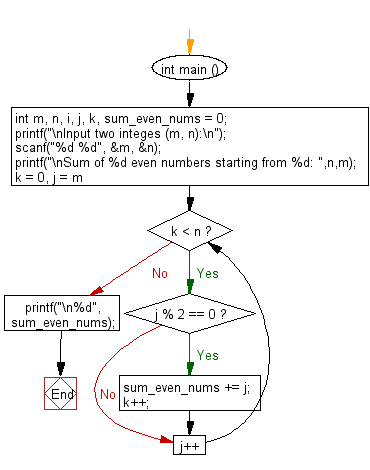﻿ C Program: Sum of n even numbers starting from m - w3resource

# C Exercises: Sum of n even numbers starting from m

## C Basic Declarations and Expressions: Exercise-122 with Solution

Write a C program that reads two integers m, n and computes the sum of n even numbers starting from m.

Sample Solution:

C Code:

``````#include <stdio.h>

int main () {
int m, n, i, j, k, sum_even_nums = 0;

// Prompt user for input
printf("\nInput two integers (m, n):\n");

// Read two integer values 'm' and 'n' from user
scanf("%d %d", &m, &n);

// Print a message indicating what the program will do
printf("\nSum of %d even numbers starting from %d: ",n,m);

// Loop to find and sum 'n' even numbers starting from 'm'
for (k = 0, j = m; k < n; j++) {

// Check if 'j' is even
if (j % 2 == 0) {
sum_even_nums += j; // Accumulate even numbers
k++; // Increment the count of even numbers found
}
}

// Print the sum of even numbers
printf("\n%d", sum_even_nums);

return 0; // End of program
}
``````

Sample Output:

```Input two integes (m, n):
20
60

Sum of 60 even numbers starting from 20:
4740
```

Flowchart:C programming Code Editor:

What is the difficulty level of this exercise?

Test your Programming skills with w3resource's quiz.

﻿